DOUBLE ACTION HYDRAULIC INTENSIFIER.
Roman N. Tunkel
Ingersoll-Rand Co., Waterjet System Division,
Farmington Hills, MI, U.S.A.
ABSTRACT
To provide a continuous flow of liquid it is necessary to create ultra high pressure in the line of the orifice in a range of 300-400 MPa. In most applications the Double Action Hydraulic Intensifier (DAHI) was used to develop this ultra high pressure. In order to calculate the parameters of DAHI, it is necessary to know the dependency of viscosity on pressure, the influence of liquid compressibility, the amount of useless space in the high pressure cylinders, the deformation of the wall of the hydraulic and high pressure cylinders, etc.
This paper represents a theoretical investigation of the mathematical model for the determination of several different parameters of the DAHI, including some parameters of the water stream and the cutting or drilling material. The semi-empirical equation for the definition of the total cycle time based on the superseding and switch time was obtained. It was shown that the acceleration phase has a negligible duration, compared to the superseding phase and switch time for the main hydraulic valve is more substantial for the total cycle time formatting. Finally, new equations for defining the parameters of the DAHI were reached.
The advantage of the new method is shown. By using these equations, solutions with high accuracy are obtained. The results of a theoretical investigation were compared to the experimental data and good correlation was found.
1. INTRODUCTION
The intensifier pump is used for creating ultra high pressure in liquids, for example in water, which is used in most of cases for cutting systems . For the creation of continuous flow of the liquid which is moving with ultrasonic speed (600-900 m/sec) it is necessary to create ultra high pressure at the line or the orifice in the range of 300-400 MPa (45,000-60,000 psi).
To develop this ultra high pressure in most applications, the Double Action Hydraulic Intensifier (DAHI) which transfers hydraulic, relatively low pressure, to ultra high water pressure, was used. In Fig.1 the basic scheme of a jet cutting unit is shown, which uses a pressure multiplier made up of two high pressure "plunger pumps", driven by a "linear hydraulic motor".
The two pumps made up of the high pressure cylinders and plungers, suction valves and discharge valves are mounted in parallel being countertime driven by linear hydraulic motor consisting of hydraulic cylinder and piston. The seals between high pressure plunger pumps and hydraulic cylinder are playing a very important role. To lower the pressure pulsations occurring during reversing the hydraulic piston the attenuator is typically mounted on the pressing line from where nozzle is supplied.
2. MODEL #1
Consider the calculation of the parameters of DAHI as a mechanical system. As a mechanical system, DAHI consist of just one movement element - the piston/plungers, which moves under total (resulting) force. This force quickly  changes from zero until a certain value and then remains constant for a relatively long time (during most of the cycle). According to that let's divide the cycle of the movement of the piston-plungers into 2 phases. Will call the first "the acceleration phase" and the second "the superseding phase". The acceleration phase is a phase of movement when water in the cylinders is still compressed and water pressure is still growing. The duration of this phase depends on mass of the piston and the multiplication ratio. The superseding phase is the phase when high water pressure becomes stabilized (reaches its maximum) and the system is balanced. The duration of this phase depends on the orifice side and geometrical parameters of the high pressure cylinder (diameter of the cylinder and stroke). Also assume that the friction between moving parts and layers of liquid is zero, the deformation of the wall of the hydraulic and high pressure cylinders is negligible. Then we will calculate the movement of the piston from the start (wall contact position) and until it reaches the proximity switch; we will not calculate any dynamic effects on the water (open/closing effects); we will not calculate the thermodynamic effects; compressibility of the water will be included in the calculations as a coefficient " comp".
For this relatively simple model, the movement is easily described by Newton's first law: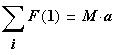whereis the mass of the piston, 2 plungers
and the oil up-front of the piston and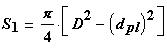The total force for the acceleration phase is superposition of the acceleration and
disacceleration forces, which in our case are (assume friction force is zero):and consider thatobtainwhere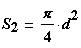Assume that the pressure in hydraulic and high water pressure cylinders
or lines changes as linear  as shown on Fig.2 and Fig.3. For these linear
dependencies and for the conditions and we have for pressuresandNotice, thatwhere rat is multiplication ratioAfter that, using these equations, and (1), and (2), we will combine the equation of movement for the acceleration phase:And after some calculation, we receive the integral for the duration of the acceleration phase (3):Using boundary conditions (t0 =0, v0 =0) finished integration and, finally, the duration of the acceleration phase was received (4):where coefficient k m is:Look over the results and build a plot for duration of the acceleration phase. For our assumptions, which are describe above, the duration of the acceleration phase has a negligible value - less then 0.5 millisec. Now, let's calculate the duration of the superseding phase. Remember that the superseding phase is the phase when high water pressure is stabilized and the system is balanced and the piston speed is a constant. Duration of this phase depends on the orifice side (flow) and volume of the high pressure cylinder (5):Because:andAfter some transformation of
(6) the integral for the duration
of the superseding phase, we
will obtain:Duration of the total cycling time for DAHI (T ) could be found by equation: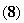where:- is a switch time for the main hydraulicc valve, approx. 100 msec.
Let's compute these equations and build the plot. For comparison, we will use some experimental data, which was received on the production DAHI. As we can see on Fig.5 , the experimental data and calculation is a better match for each other if we assume that Kor equals 0.75.
Finally, we can assume (see Fig.4 ), the acceleration phase has a negligible duration, compared with the superseding phase ( Fig.5 ) and even switch time for main hydraulic valve more substantial for total cycle time formatting (8).
Therefore, we can ignore the acceleration phase
and calculate a total cycle time based on the
superseding and switching time only (9):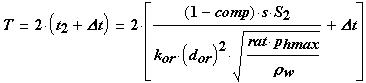where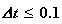anddefine by (21).
Let's again compare the result of calculation by equation (9) with experimental data. Remember that we are calculating simple model in assumption that the friction between moving parts and layers of liquid is zero, the deformation of the wall of the hydraulic and high pressure cylinders is negligible. Remember also, that thermodynamic and dynamic effects in the water (open/closing effects) were not included in this calculation. However, even for that very simple model we received good agreement between experimental data and calculated results (Fig.6, Fig.7).
3. MODEL #2
For a more accurate approach to calculation of the parameters of DAHI, it is necessary to know the dependence of the viscosity changes versa pressure, the influence of liquid compressibility, the volumes of useless space of the high pressure cylinders, the deformation of the wall of the hydraulic and high pressure cylinders etc.  . Also, it is necessary to establish some parameters of the water stream and the cutting or drilling material. We will use the same schema of the DAHI which is shown above ( Fig.1 ). For this computation of the DAHI, let's establish several additional dimensionless parameters, using the diameter of the plunger as the base (10):
K pl = L/d pl
- is plunger ratio;
Kcl =(d s -d pl )/2*d
- is clearance ratio.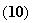Ks = L s /d pl
- is seal ratio;
Let's calculate the volume of the high pressure cylinder (V hpc ) of the DAHI which is necessary to have in order to reach the required flow through orifice. In this case, (ultra high pressure) it is necessary to consider the compressibility of the liquid, the useless space of the high pressure cylinders and the deformation of the plunger diameter (d2pl) or wall (11):where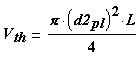- is the theoretical (maximum) volume of lliquid which could be supplied in line during one stroke of the plunger.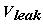- is volume of the leakage through the cleearance between the plunger and sealing per one stroke of the plunger.- is reduction of the total volume as a reesult of the compressibility of the liquid per one stroke of the plunger.- is additional volume as a result of the deformation of the wall of the cylinders per one stroke of the plunger.
Because in the worst case the plunger could be excentrically related to the seal or hydraulic cylinder and we can estimate the leakage volume by using a well known equation . In the case of plunger deformation, the leakage volume could bedefined by the formula (13):where:- is radial clearance between seal and plunger;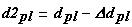- is deformed plunger diameter;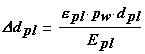- is plunger deformation. The non-deformaated diameter of the plunger is:- is non-deformated plunger diameter;
For 420 MPa (60,000 psi) pressure deformation of the plunger could reach 0.50 - 0.75 micrometers. The metal to metal seal surfaces are required a clearance of about 3 - 5 micrometers. This means that, for this case, the deformation of the plunger could reach 10% of the regular clearance.
The reduction of the total volume as a result of the
compressibility of the liquid is defined by a formula (15):where: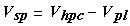- is volume of the high pressure cylinder which not fillet by plunger and always stays with the liquid.
The additional volume as a result of the deformation of the
wall of the cylinders is defined by a formula (16):
Notice, that this volume is also changed by pressure.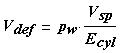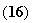Finally, using equations (10) - (16) , the expression for the volume of the high pressure cylinder are combined (17):Make some transformations, and using substitution for ratios K pl , K s , and K cl (10), we obtain an equation for the non-deformated diameter of the plunger d pl :
This equation (18), which includes real leakage and deformation of the parts of DAHI and real properties of the water under high pressure gives us the possibility to select desirable geometrical parameters for a new design of DAHI.The equation (17) is also possible to use for the definition or prediction of the cycle time of DAHI, if main geometrical parameters of the two high pressure "plunger pumps" and "linear hydraulic motor" are known.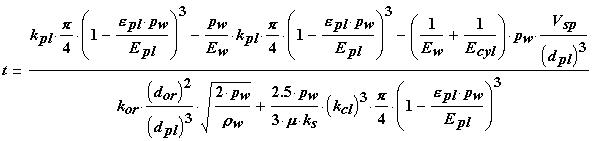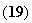For the calculation of two unknown parameters of the water properties in the equations (18), (19) the experimental data were used. The author himself created the polynomial regression equations for dynamic viscosity and density of the water under high pressure and different temperatures (20), (21):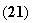The equations (20) are authorized polynomial regression equations for the dynamic viscosity of the water for high pressure (kg/m 2 ) and constant temperature of 0, 30, 75 C degrees, by source . The equations (21) are authorized polynomial regression equations for a density of the water under pressure (atm ), by source [ 1 ];
Results of the calculations made by equations (19), (20), (21) are compared with the experimental data for cycle time of the production of DAHI, represented in Fig.8 and Fig.9
4. ANALYSIS OF RESULTS
We can see that the result of these calculations for such very different models are very close to each other and to the experimental data. In actuality, model #2 uses the approach when superseding, switch and acceleration time is based on or estimated by time of flow through the orifice. Notice, that for model #1 the total cycle time depends on water pressure, compressibility, geometrical parameters, such as stroke of the piston, diameter of the hydraulic piston and plunger, diameter of the orifice and coefficient of orifice.
Also notice, that for model #2 the total cycle time depends on the same parameters along with clearance between the plunger and seal, on properties of the liquid and material properties of parts such as plunger, hydraulic cylinder.
However, even for a very simple model, such as model #1, we received good agreement between the experimental data and the calculated results.
5. CONCLUSIONS
1. Since the acceleration phase of DAHI has a negligible duration, the equation for the total cycle time, based solely on the superseding and switching time (9) was obtained and good agreement with experimental data was shown.

2. The more accurate mathematical model of DAHI, model #2 (19) which includes real leakage and deformation of the parts of DAHI and real properties of the water under high pressure, gives us the possibility to predict the cycle time for the existing design of DAHI and /or select desirable geometrical parameters for a new design of DAHI.

3. It is theoretically proven by two different ways that the total cycle time of DAHI is proportional to the value of water pressure, compressibility of the water, plunger diameter and stroke, but inversely proportional to the value of flow rate or orifice diameter.

4. Within the range of the performed experiments, the results of analysis show that a simple mathematical model of DAHI should account for the influence of main geometrical parameters of DAHI and that the duration of acceleration phase is
neglected compared to the duration of superseding phase, or even switching time for the main hydraulic valve.

5. A mathematical pattern is presented for studying the operation of very high pressure plunger pumps by taking into account
the fluid compressibility.

6. Both theoretical equations are compared with the experimental results and a good agreement is observed in the field of pump rated pressure and high values for the attenuator volume.
ACKNOWLEDGMENTS
The author gratefully acknowledge significant contributions from Jose Munoz for actively supporting the research and development over many years.
REFERENCES
1. Bridgemen, P.V., "Physics of the high pressure," 1953 by Machinostroenie, Moscow, USSR.

2. Chalmers, E.J., "Pressure Fluctuation and operating efficiency of intensifier pumps," Proceeding of the 7th American Water Jet conference , pp.327-337, Water Jet Technology Association, 1993, Seattle, USA.

3. Singh,P.J., Benson, D., "Development of phased intensifier for waterjet cutting," Proceeding of the 11th International Conference, pp.305-319, Jet Cutting Technology, Scotland, 1992.

4. Susan-Resiga, R., "Attenuator's Volume influence on high pressure's pulsations in a jet cutting unit," Proceeding of the 11th International Conference, pp.37-47, Jet Cutting Technology, Scotland, 1992.

5. Vereschagin, L.F., Semertchan, A.A., Firsov, A.I., "Studies of Hydrodynamics of a liquid jet outflowing from under Pressure up to 4500 atm.," ZhTPh, 26 (11), 2570-2579 pp., 1956;

6. White, F.M., "Viscous Fluid Flow," 1974 by McGraw-Hill, Inc., USA.

7. Zaring, K., "Advanced abrasive-waterjet hardware and cutting performance," Proceeding of the 5th American Water Jet conference, pp.473-483, Water Jet Technology Association, 1989, Canada.
NOMENCLATURE- a hydraulic cylinder diameter;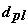- the diameter of the undeformed plunger;- the diameter of the deformed plunger;
d s
- a diameter of the seal;
L
- a length of the cylinder;
L s
- the length of the seal between hydraulicc and high pressure cylinder;- a width of the piston;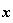- a displacement of the piston;
v
- a speed of the piston;
s
- a stroke hydraulic piston or high pressuure plunger;
a
- an acceleration;
M
- a mass of the movement parts;- a works piston area of the hydraulic cyllinder;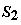- a works plunger area;
F h
F w
- a force developed by hydraulic pressure;
- a force developed by water pressure;
F(1)
- a total movement force;
f
- a frequency of the reverses (number of work cycle per second);
r w
- a density of water;
r oil
- a density of oil;
p h
- a hydraulic line pressure;
p w
- a water pressure;
Q
- a consumption of the high pressure liquid (water) through orifice;
d or
k or
- a coefficient of the orifice;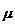- a dynamic viscosity of the water;- is Poisson coefficient for the plunger material;
E pl
- a module elasticity for the plunger material;
E w
- a module elasticity for the liquid;
E cyl
- a module elasticity for the high pressurre cylinder material;- a duration of the acceleration phase;- a duration of the superseeding phase;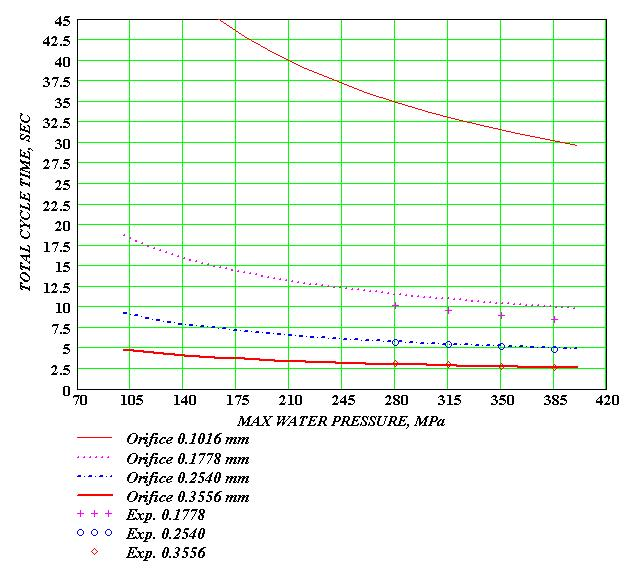O
R
I
F
I
C
E,

mm
O
R
I
F
I
C
E,

mm
LINE PRESSURE, MPa
LINE PRESSURE, MPa